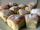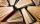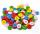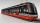# Football league

In the 2nd football league plays each of the 16 participants with each opponent twice. For every victory gets 3 points, 1 point for a draw, a defeat none. After the competition was team with 54 points and 12 defeats at 9th place. How many times have won?

Result

x =  18

#### Solution:

2(16-1) = x + r + 12
54 = 3x + r

r+x = 18
r+3x = 54

r = 0
x = 18

Calculated by our linear equations calculator.

Leave us a comment of example and its solution (i.e. if it is still somewhat unclear...):

Showing 0 comments:Be the first to comment!#### To solve this verbal math problem are needed these knowledge from mathematics:

Do you have a system of equations and looking for calculator system of linear equations?

## Next similar examples:

1. Three friendsThe three friends spent 600 KC in a teahouse. Thomas paid twice as much as Paul. Paul a half less than Zdeněk. How many each paid?
2. MushroomsEva and Jane collected 114 mushrooms together. Eve found twice as much as Jane. How many mushrooms found each of them?
3. Three brothersThe three brothers have a total of 42 years. Jan is five years younger than Peter and Peter is 2 years younger than Michael. How many years has each of them?
4. Three daysDuring the three days sold in stationery 1490 workbooks. The first day sold about workbooks more than third day. The second day 190 workbooks sold less than third day. How many workbooks sold during each day?
5. Linear systemSolve this linear system (two linear equations with two unknowns): x+y =36 19x+22y=720
6. DonutsIn the dining room at one table sit Thomas, Filip and Martin. Together they eat 36 donuts. How many donuts eaten everyone, when Filip ate twice as much as Thomas and Martin even half over Filip?
7. Pages of bookLenka calculated that if every day to read 16 pages of the book, read a book a day earlier than had read only 14 pages a day. How many pages has this book?
8. Magic numberThe number 135 split to two addends so that one addend was 30 greater than 2/5 the addend.
9. Hotel roomsIn the 45 rooms, there were 169 guests, some rooms were three-bedrooms and some five-bedrooms. How many rooms were?
10. EquationsSolve following system of equations: 6(x+7)+4(y-5)=12 2(x+y)-3(-2x+4y)=-44
11. ButtonsShirt has 6 buttons, blouse has 4 buttons. All buttons are 176. Shirts and blouses are together 36. How many are the shirts and blouses?
12. TreesAlong the road were planted 250 trees of two types. Cherry for 60 CZK apiece and apple 50 CZK apiece. The entire plantation cost 12,800 CZK. How many was cherries and apples?
13. Two numbersWe have two numbers. Their sum is 140. One-fifth of the first number is equal to half the second number. Determine those unknown numbers.
14. Waiting roomIn the waiting room are people and flies. Together they have 15 heads and 50 legs (fly has 6 legs). How many people and flies are in the waiting room?
15. TramIn the three-part tram went 206 passengers in front of others, 226 after others and in the middle half of all. How many passengers went in tram total?
16. TheatroTheatrical performance was attended by 480 spectators. Women were in the audience 40 more than men and children 60 less than half of adult spectators. How many men, women and children attended a theater performance?
17. Equations - simpleSolve system of linear equations: x-2y=6 3x+2y=4